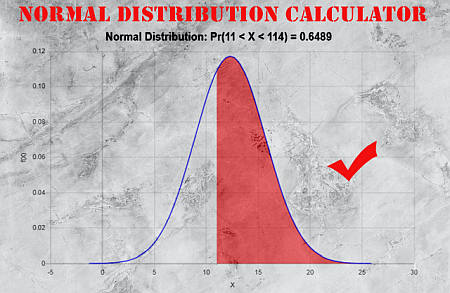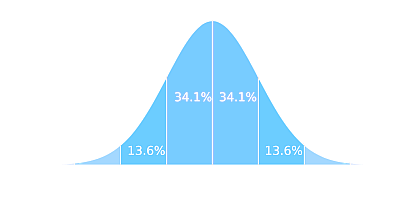# Normal Probability Calculator for Sampling Distributions

Instructions: This Normal Probability Calculator for Sampling Distributions will compute normal distribution probabilities for sample means $$\bar X$$, using the form below. Please type the population mean ($$\mu$$), population standard deviation ($$\sigma$$), and sample size ($$n$$), and provide details about the event you want to compute the probability for (for the standard normal distribution, the mean is 0 and the standard deviation is 1):Population Mean ($$\mu$$)Population St. Dev. ($$\sigma$$)Sample Size ($$n$$)
Two-Tailed:
$$\le \bar X \le$$
Left-Tailed:
$$\bar X \le$$
Right-Tailed:
$$\bar X \ge$$

When a sequence of normally distributed variables $$X_1, X_2, ...., X_n$$ is averaged, we get the sample mean

$\bar X = \frac{1}{n}\sum_{i=1}^n X_i$

Since any linear combination of normal variables is also normal, the sample mean $$\bar X$$ is also normally distributed (assuming that each $$X_i$$ is normally distributed). The distribution of $$\bar X$$ is commonly referred as to the sampling distribution of sample means .

Another name you will see the normal distribution referred about is the gaussian distribution, or the bell-shaped distribution.### How do you calculate sampling distribution?

Assuming that $$X_i \sim N(\mu, \sigma^2)$$, for all $$i = 1, 2, 3, ...n$$, then $$\bar X$$ is normally distributed with the same common mean $$\mu$$, but with a variance of $$\displaystyle\frac{\sigma^2}{n}$$.

This tells us that $$\bar X$$ is also centered at $$\mu$$ but its dispersion is less than that for each individual $$X_i$$. Indeed, the larger the sample size, the smaller the dispersion of $$\bar X$$.

### The Normal Distribution formula

The normal distribution formula is a relatively difficult one, that is not one you will be handling manually. The formula is:

$f(x)=\frac{1}{\sigma {\sqrt {2\pi }}} e^{-{\frac {1}{2}}\left({\frac {x-\mu }{\sigma }}\right)^{2}}$

### The sampling normal distribution formula

The key when working with sampling distributions is to use the fact that if $$\mu$$ is the mean of the population and $$\sigma$$ is the standard deviation of the population, then

$\displaystyle \frac{\bar X - \mu}{\sigma}$

has a standard normal distribution. This is crucial, because we can use this to reduce all sampling distributions into standard normal probability calculations.

In simple terms, what you are doing is reducing the calculation of any normal distribution probability into the calculation of z-scores.

By reducing all normal distribution calculations to working with z-scores, all you need to have is one standard normal table, where to find the z-values, or a tool like this calculator or Excel.

### What is the mean of the sampling distribution

The mean of sampling distributions, $$\mu(\bar X)$$, is the same as the underlying mean of the distribution $$\mu$$.

### Standard deviation of sampling distribution

Unlike the case of the mean, the standard deviation of sample means can be calculated using the formula:

$s(\bar X) = \displaystyle \frac{\sigma}{\sqrt n}$

### Calculators Related to the normal distribution

If you want to compute normal probabilities for one single observation $$X$$, you can use this calculator with $$n=1$$, or you can use our regular normal distribution calculator .

Often times you are interested in the reverse process: Given a probability, you want to find the score such as the probability to the right of that score is that given probability, for which you can use a invnorm calculator

Also, if graphical visualization is what you need, you can try directly our normal distribution graph creator.

Also, in order to assess if a sample comes from an actual normal distribution, you can use a normal probability plot, and see the pattern obtained. If it looks fairly linear, it indicates the sample likely came from a normally distributed propulation.### Example:

Question: Consider a normal distribution where the population mean is 12, and the population standard deviation is 3.4. Assume you take samples of size n = 16. What is the probability for the sample means to be in the interval (11.3, 12.4)?

Solution:

The following are the population mean $$(\mu)$$, population standard deviation $$(\sigma)$$ and sample size $$(n)$$ provided:

 Population Mean $$(\mu)$$ = $$12$$ Population Standard Deviation $$(\sigma)$$ = $$3.4$$ Sample Size $$(n)$$ = $$16$$ Event to compute its probability = $$11.3 \leq \bar X \leq 12.4$$

We need to compute $$\Pr(11.3 \leq \bar X \leq 12.4)$$. The corresponding z-values needed to be computed are:

$Z_{lower} = \frac{X_1 - \mu}{\sigma/\sqrt{n}} = \frac{ 11.3 - 12}{ 3.4/\sqrt{16}} = -0.82$ $Z_{upper} = \frac{X_2 - \mu}{\sigma/\sqrt{n}} = \frac{ 12.4 - 12}{ 3.4/\sqrt{16}}= 0.47$

Using the properties of the normal distribution, if $$X ~ N(\mu, \sigma)$$, then the variables $$Z_{lower} = \displaystyle \frac{X_1 - \mu}{\sigma/\sqrt{n}}$$ and $$Z_{upper} = \displaystyle \frac{X_2 - \mu}{\sigma/\sqrt{n}}$$ have a standard normal distribution. Therefore, the probability is computed as:

$\begin{array}{ccl} \Pr(11.3 \leq \bar X \leq 12.4) & = & \Pr\left(\displaystyle \frac{ 11.3 - 12}{ 3.4 / \sqrt{ 16}} \leq \frac{ \bar X - 12}{ 3.4 / \sqrt{ 16}} \leq \frac{ 12.4 - 12}{ 3.4 / \sqrt{ 16}}\right) \\\\ \\\\ & = & \displaystyle\Pr\left(\frac{ 11.3 - 12}{ 3.4 / \sqrt{ 16}} \leq Z \leq \frac{ 12.4 - 12}{ 3.4 / \sqrt{ 16}}\right) \\\\ \\\\ & = & \displaystyle \Pr\left(-0.82 \leq Z \leq 0.47\right) \\\\ \\\\ & = & \displaystyle \Pr\left(Z \leq 0.47\right) - \Pr\left(Z \leq -0.82\right) \\\\ \\\\ & = & 0.681 - 0.2051 \\\\ \\\\ & = & 0.4759 \end{array}$

Therefore, based on the information provided, it is concluded that $$\Pr(11.3 \leq \bar X \leq 12.4) = 0.4759$$.